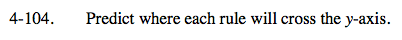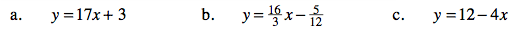### Home > CAAC > Chapter 4 > Lesson 4.2.4 > Problem4-104

4-104.The y-intercept occurs when x = 0.

y-intercept = (0, 3)

If y = mx + b, b = the y-intercept.

What is b in this equation?

$-\frac{5}{12}$

y intercept =

$\left(0,-\frac{5}{12}\right)$

Use the hints from parts (a) and (b).